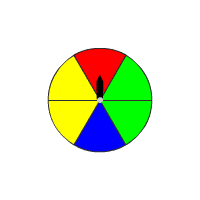# Using Expected Values to Make Decisions

The expected value of a random variable can be used to make decisions, for example about whether to place a bet or play a game.

Example:

At a school fair, you are given a number of tokens. In one stall at the fair, there is a spinner with $6$ sectors. If the spinner lands on a red sector, you win $2$ tokens. If you land on a blue sector, you win $5$ tokens. If you land on any other sector, you lose $1$ token.

Are you better off playing this game or not?The spinner has $6$ sectors and each is an equally likely possibility.

Sample space is {red sector, blue sector, $4$ other sectors}

Write the probability distribution for a single spin of spinner and the amount of tokens you win.

$\begin{array}{|cccc|}\hline x=\text{color}& \text{Red}& \text{Blue}& \text{Others}\\ P\left(x\right)& \frac{1}{6}& \frac{1}{6}& \frac{4}{6}\\ \text{Tokens}& 2& 5& -1\\ \hline\end{array}$

Use the weighted average formula.

$\begin{array}{l}E\left(x\right)=2\cdot \frac{1}{6}+5\cdot \frac{1}{6}+\left(-1\right)\cdot \frac{4}{6}\\ =\frac{2}{6}+\frac{5}{6}-\frac{4}{6}\\ =\frac{3}{6}\text{\hspace{0.17em}}\text{\hspace{0.17em}}\text{or}\text{\hspace{0.17em}}\text{\hspace{0.17em}}0.5\end{array}$

So, the expected value is $0.5$ tokens, which is positive. In other words, on average, you get $0.5$ token per spin, so you are better off playing this game.